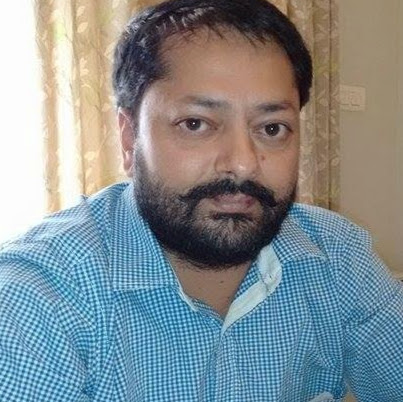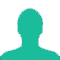# Ten different letters of alphabet are given, words with 5 letters are formed from these given letters. Then, the number of words which have at least one letter repeated is:

A. 69760

B. 30240

C. 99748

D. 42386

### Solution(By Examveda Team)

Number of words which have at least one letter replaced,
= Total number of words - total number of words in which no letter is repeated.
= 10516P5
= 100000 − 30240
= 69760

This Question Belongs to Arithmetic Ability >> Permutation And Combination

1.16p5 is incorrect 10p5 should be there and value of 10p5 is 30240.

2.Why it is not 10^5 -10p5

3.10^5- 10p5 is correct solution

4.Correct solution is 10^5- 10p5

5.why we have taken 16p5 and why not 10p5

Related Questions on Permutation and Combination